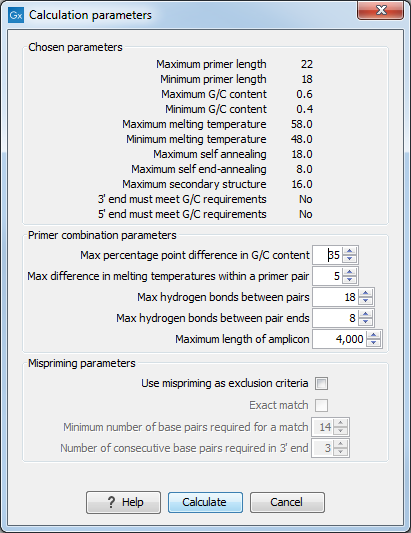## When both forward and reverse regions are defined

If both a forward and a reverse region are defined, primer pairs will be suggested by the algorithm.

After pressing the Calculate button a dialog will appear (see figure 34.8).Figure 34.8: Calculation dialog for PCR primers when two primer regions have been defined.

Again, the top part of this dialog shows the parameter settings chosen in the Primer parameters preference group which will be used by the design algorithm. The lower part again contains a menu where the user can choose to include mispriming of both primers as a criteria in the design process (see When a single primer region is defined). The central part of the dialog contains parameters pertaining to primer pairs. Here three parameters can be set:

• Maximum percentage point difference in G/C content - if this is set at e.g. 5 points a pair of primers with 45% and 49% G/C nucleotides, respectively, will be allowed, whereas a pair of primers with 45% and 51% G/C nucleotides, respectively will not be included.
• Maximal difference in melting temperature of primers in a pair - the number of degrees Celsius that primers in a pair are all allowed to differ.
• Max hydrogen bonds between pairs - the maximum number of hydrogen bonds allowed between the forward and the reverse primer in a primer pair.
• Max hydrogen bonds between pair ends - the maximum number of hydrogen bonds allowed in the consecutive ends of the forward and the reverse primer in a primer pair.
• Maximum length of amplicon - determines the maximum length of the PCR fragment.